# 前言#

Go 语言的官方包 imageimage/color 定义了非常多的类型，涵盖了很多的图像处理基础内容，本文简单介绍这些库中的基本概念和使用方法。

# 常见类型介绍#

## Colors#

Colors 是一个接口，它的代码如下：

``````type Color interface {
// RGBA returns the alpha-premultiplied red, green, blue and alpha values
// for the color. Each value ranges within [0, 0xffff], but is represented
// by a uint32 so that multiplying by a blend factor up to 0xffff will not
// overflow.
//
// An alpha-premultiplied color component c has been scaled by alpha (a),
// so has valid values 0 <= c <= a.
RGBA() (r, g, b, a uint32)
}
``````

`Color` 的 rgba 值都是经过了 alpha 预乘的。

`Color` 定义的 rgba 值类型是 `uint32`，但是它存储的颜色是 16 位色深，也就是其最大值为 65535，这样做为了兼容一些算法，以防止在某些乘法运算中出现溢出1

## RGBA#

`image/color` 包同样定义了很多实现了 `Color` 接口的颜色类型，例如 RGBA，它的代码如下：

``````type RGBA struct {
R, G, B, A uint8
}
``````

## Model#

Model 是一个可以将 `Colors` 转化为其他 `Colors` 的接口，这个转化可能是有损失的。例如，`GrayModel` 可以将任何颜色转换为去饱和的灰色 Gray`Palette` 可以将任意颜色转化为有限的调色板中的一种颜色。

``````type Model interface {
Convert(c Color) Color
}

type Palette []Color
``````

## Points#

``````type Point struct {
X, Y int
}
``````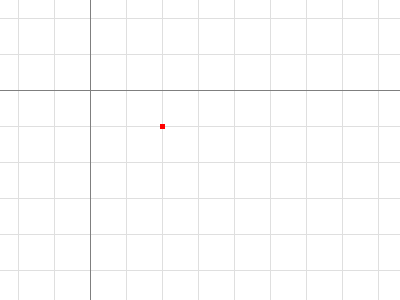``````p := image.Point{2, 1}
``````

## Rectangles#

``````type Rectangle struct {
Min, Max Point
}
``````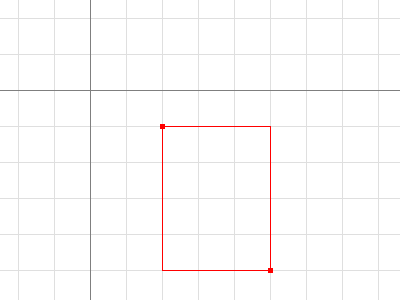``````r := image.Rect(2, 1, 5, 5)
// Dx 和 Dy 返回一个矩形的宽和高
fmt.Println(r.Dx(), r.Dy(), image.Pt(0, 0).In(r)) // prints 3 4 false
``````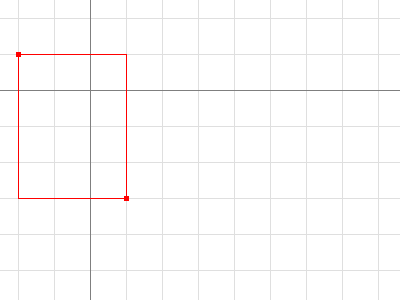``````r := image.Rect(2, 1, 5, 5).Add(image.Pt(-4, -2))
fmt.Println(r.Dx(), r.Dy(), image.Pt(0, 0).In(r)) // prints 3 4 true
``````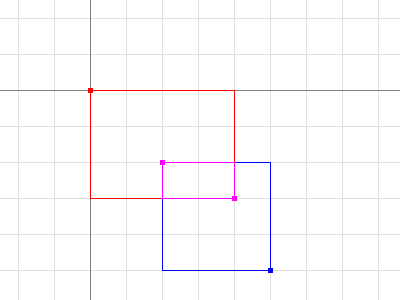``````r := image.Rect(0, 0, 4, 3).Intersect(image.Rect(2, 2, 5, 5))
// Size 返回一个矩形的宽和高，作为一个点
fmt.Printf("%#v\n", r.Size()) // prints image.Point{X:2, Y:1}
``````

`Point` 和矩形 `Rectangles` 以值传递的方式返回值。将一个 `Rectangle` 作为 argument 传入一个函数相当于传入俩个 `Point` 或四个 `int`

## Images#

``````type Image interface {
// ColorModel returns the Image's color model.
ColorModel() color.Model
// Bounds returns the domain for which At can return non-zero color.
// The bounds do not necessarily contain the point (0, 0).
Bounds() Rectangle
// At returns the color of the pixel at (x, y).
// At(Bounds().Min.X, Bounds().Min.Y) returns the upper-left pixel of the grid.
// At(Bounds().Max.X-1, Bounds().Max.Y-1) returns the lower-right one.
At(x, y int) color.Color
}
``````

``````b := m.Bounds()
for y := b.Min.Y; y < b.Max.Y; y++ {
for x := b.Min.X; x < b.Max.X; x++ {
doStuffWith(m.At(x, y))
}
}
``````

`Image` 的实现并不一定基于内存切片中的像素数据。例如，`Uniform` 表示一个具有巨大边界和统一颜色的图像，其内存就表示该颜色。

``````type Uniform struct {
C color.Color
}
``````

``````type RGBA struct {
// Pix holds the image's pixels, in R, G, B, A order. The pixel at
// (x, y) starts at Pix[(y-Rect.Min.Y)*Stride + (x-Rect.Min.X)*4].
Pix []uint8
// Stride is the Pix stride (in bytes) between vertically adjacent pixels.
Stride int
// Rect is the image's bounds.
Rect Rectangle
}
``````

``````m := image.NewRGBA(image.Rect(0, 0, 640, 480))
m.Set(5, 5, color.RGBA{255, 0, 0, 255})
``````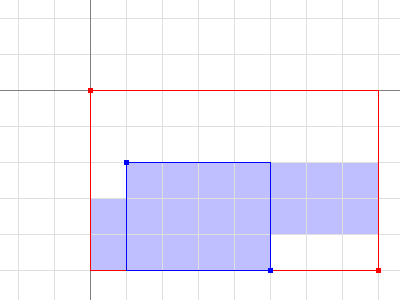``````m0 := image.NewRGBA(image.Rect(0, 0, 8, 5))
m1 := m0.SubImage(image.Rect(1, 2, 5, 5)).(*image.RGBA)
fmt.Println(m0.Bounds().Dx(), m1.Bounds().Dx()) // prints 8, 4
fmt.Println(m0.Stride == m1.Stride)             // prints true
``````

## Image Formats#

Go 语言标准库支持多种常见的图像格式，例如 GIF、JPEG 和 PNG。如果你知道源图像的文件格式，可以直接使用 io.Reader 进行解码。

``````import (
"image/jpeg"
"image/png"
"io"
)

// convertJPEGToPNG converts from JPEG to PNG.
func convertJPEGToPNG(w io.Writer, r io.Reader) error {
img, err := jpeg.Decode(r)
if err != nil {
return err
}
return png.Encode(w, img)
}
``````

``````import (
"image"
"image/png"
"io"

_ "image/jpeg"
)

// convertToPNG converts from any recognized format to PNG.
func convertToPNG(w io.Writer, r io.Reader) error {
img, _, err := image.Decode(r)
if err != nil {
return err
}
return png.Encode(w, img)
}
``````

# 参考链接#

https://go.dev/blog/image